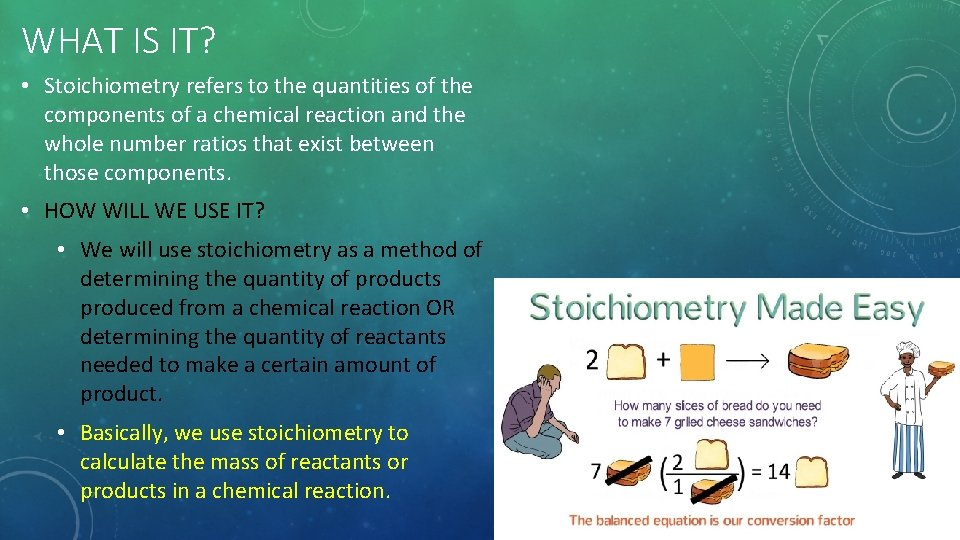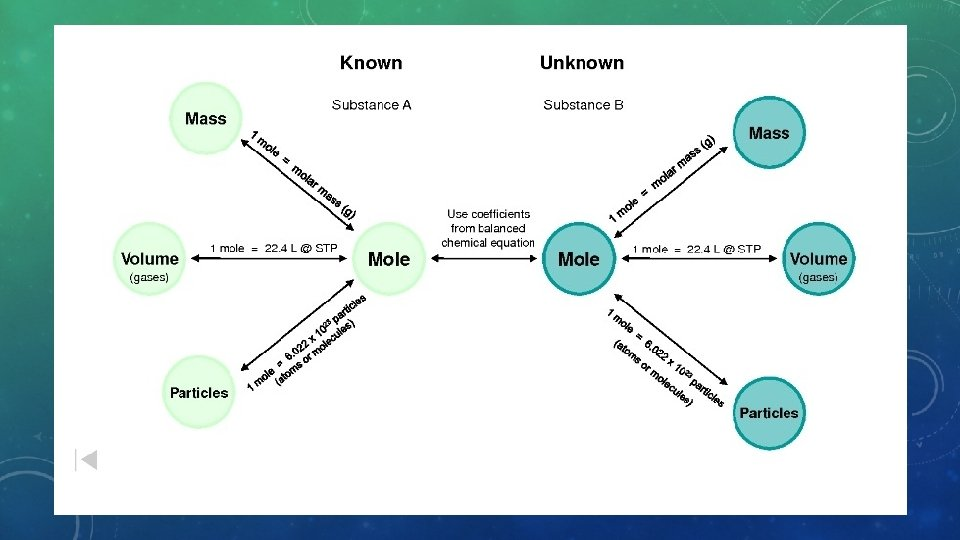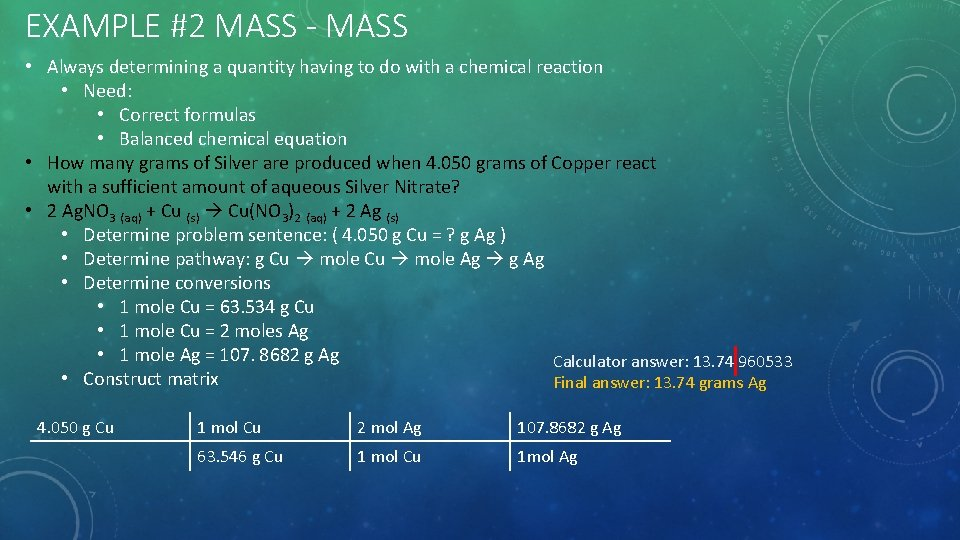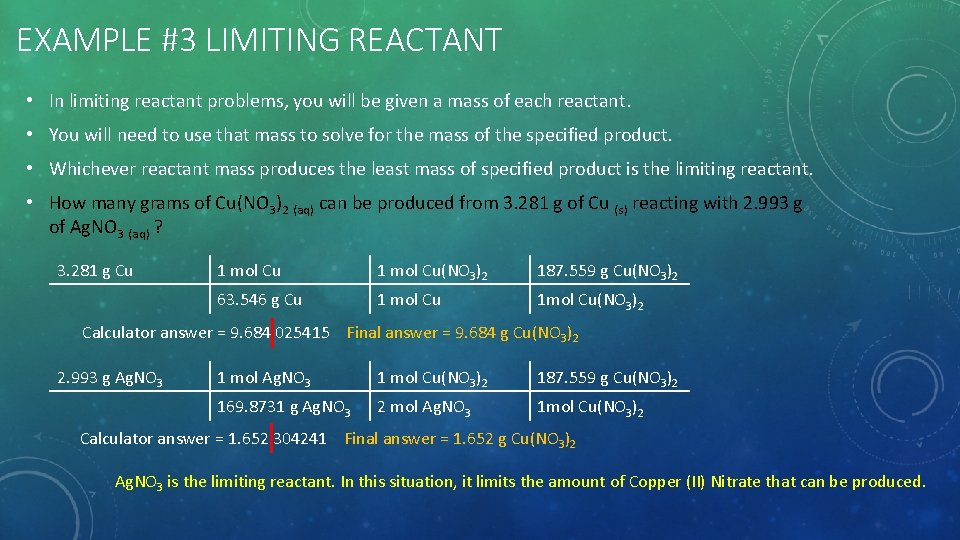# STOICHIOMETRY WHAT IS IT Stoichiometry refers to the

• Slides: 6STOICHIOMETRYWHAT IS IT? • Stoichiometry refers to the quantities of the components of a chemical reaction and the whole number ratios that exist between those components. • HOW WILL WE USE IT? • We will use stoichiometry as a method of determining the quantity of products produced from a chemical reaction OR determining the quantity of reactants needed to make a certain amount of product. • Basically, we use stoichiometry to calculate the mass of reactants or products in a chemical reaction.EXAMPLE #1 MASS - MOLE • How many moles is equivalent to 5. 274 grams of Sodium Hydroxide? • Start with simplifying the question (Problem sentence). What is it actually asking you to figure out? • 5. 274 g Na. OH = ? moles Na. OH • Then, figure out the conversions you’ll need to make to solve the problem (Pathway). • g Na. OH moles Na. OH • Determine the actual conversions that you’ll need. • 1 mole Na. OH = 39. 9971 grams Na. OH This is just the molar mass. The molar mass is ALWAYS the mass of ONE mole. • Do we need any more conversions? • Set up the matrix and solve the problem. • Draw a horizontal line. • The number of arrows in your pathway are the number of vertical lines in your matrix. 5. 274 g Na. OH 1 mol Na. OH 39. 9971 g Na. OH Calculator answer = 0. 1318 59559818087 Final answer = 0. 1319 moles Na. OHEXAMPLE #2 MASS - MASS • Always determining a quantity having to do with a chemical reaction • Need: • Correct formulas • Balanced chemical equation • How many grams of Silver are produced when 4. 050 grams of Copper react with a sufficient amount of aqueous Silver Nitrate? • 2 Ag. NO 3 (aq) + Cu (s) Cu(NO 3)2 (aq) + 2 Ag (s) • Determine problem sentence: ( 4. 050 g Cu = ? g Ag ) • Determine pathway: g Cu mole Ag g Ag • Determine conversions • 1 mole Cu = 63. 534 g Cu • 1 mole Cu = 2 moles Ag • 1 mole Ag = 107. 8682 g Ag Calculator answer: 13. 74 960533 • Construct matrix Final answer: 13. 74 grams Ag 4. 050 g Cu 1 mol Cu 2 mol Ag 107. 8682 g Ag 63. 546 g Cu 1 mol Cu 1 mol AgEXAMPLE #3 LIMITING REACTANT • In limiting reactant problems, you will be given a mass of each reactant. • You will need to use that mass to solve for the mass of the specified product. • Whichever reactant mass produces the least mass of specified product is the limiting reactant. • How many grams of Cu(NO 3)2 (aq) can be produced from 3. 281 g of Cu (s) reacting with 2. 993 g of Ag. NO 3 (aq) ? 3. 281 g Cu 1 mol Cu(NO 3)2 187. 559 g Cu(NO 3)2 63. 546 g Cu 1 mol Cu 1 mol Cu(NO 3)2 Calculator answer = 9. 684 025415 Final answer = 9. 684 g Cu(NO 3)2 2. 993 g Ag. NO 3 1 mol Cu(NO 3)2 187. 559 g Cu(NO 3)2 169. 8731 g Ag. NO 3 2 mol Ag. NO 3 1 mol Cu(NO 3)2 Calculator answer = 1. 652 304241 Final answer = 1. 652 g Cu(NO 3)2 Ag. NO 3 is the limiting reactant. In this situation, it limits the amount of Copper (II) Nitrate that can be produced.